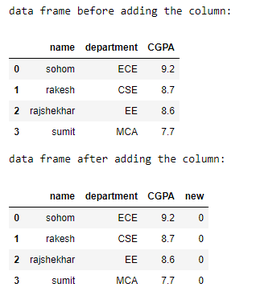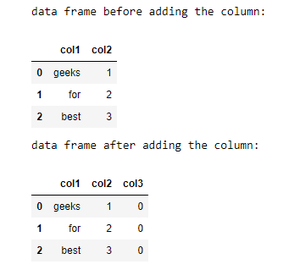Related Articles

# Add zero columns to Pandas Dataframe

• Last Updated : 11 Dec, 2020

Prerequisites: Pandas

The task here is to generate a Python program using its Pandas module that can add a column with all entries as zero to an existing dataframe.

A Dataframe is a two-dimensional, size-mutable, potentially heterogeneous tabular data.It is used to represent data in tabular form like an Excel file format. Below is the syntax for creating a dataframe in pandas. It can be imagines as a dictionary like container for series object.

Syntax:

DataFrame(data=None, index=None, columns=None, dtype=None, copy=False)

### Approach

• Import required libraries
• Create or import data
• Add a new column with all zeroes.

Example 1:

## Python3

 `# import pandas library``import` `pandas as pd`` ` `# creating dictionary of lists``dict` `=` `{``'name'``: [``"sohom"``, ``"rakesh"``, ``"rajshekhar"``, ``"sumit"``],``        ``'department'``: [``"ECE"``, ``"CSE"``, ``"EE"``, ``"MCA"``],``        ``'CGPA'``: [``9.2``, ``8.7``, ``8.6``, ``7.7``]}`` ` `# creating a dataframe``df ``=` `pd.DataFrame(``dict``)`` ` `print``(``"data frame before adding the column:"``)``display(df)`` ` `# creating a new column``# of zeroes to the``# dataframe``df[``'new'``] ``=` `0`` ` `# showing the dataframe``print``(``"data frame after adding the column:"``)``display(df)`

Output:Example 2:

## Python3

 `# import pandas library``import` `pandas as pd`` ` `# create data``data ``=` `[[``"geeks"``, ``1``], [``"for"``, ``2``], [``"best"``, ``3``]]`` ` `# creating a dataframe``df ``=` `pd.DataFrame(data, columns``=``[``'col1'``, ``'col2'``])`` ` `print``(``"data frame before adding the column:"``)``display(df)`` ` `# creating a new column with all zero entries``df[``'col3'``] ``=` `0`` ` `# showing the dataframe``print``(``"data frame after adding the column:"``)``display(df)`

Output:Attention geek! Strengthen your foundations with the Python Programming Foundation Course and learn the basics.

To begin with, your interview preparations Enhance your Data Structures concepts with the Python DS Course. And to begin with your Machine Learning Journey, join the Machine Learning – Basic Level Course

My Personal Notes arrow_drop_up